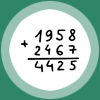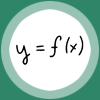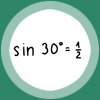Counting Addition MultiplicationVariable Function Solving equationsLine Plane ShapeSine Cosine TangentRandom variable Basic set operations DataLimits and continuity Derivative Vector